# Fear and Loathing with APL

Having heard so much about APL from Phil Trelford (who is one of the leaders in the F# community) I decided to try it out too. For the uninitiated, APL code looks every bit as mind-bending and unbelievable as scenes from Johnny Depp’s Fear and Loathing in Las Vegas.

For example, the life function below implements Conway’s game of life:

$latex life\gets\{\uparrow1 \ \omega\lor.\land3 \ 4=+/,^-1 \ \emptyset \ 1\circ.\ominus^-1 \ \emptyset \ 1\circ.\phi\subset\omega\}&s=2$

I know, right? WTF and mind blowing in equal measures…

As I was learning through Mastering Dyalog APL, I took down plenty of notes which I hope will help get you up to speed with the basics quickly.

You can try out the examples at TryAPL, just click on the “APL Keyboard” button and use the virtual keyboard to enter the symbols you need.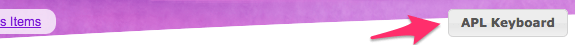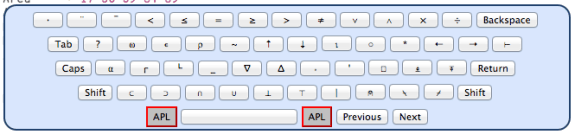## Learn APL in 10 Minutes

$latex +$ and $latex -$ does what you’d expect but multiplication and division becomes $latex \times$ and $latex \div$ (just like in maths).

$latex *$ in APL is power, i.e. $latex 7 * 3 = 7 \times 7 \times 7$

/ in APL also means something else, which we’ll come to later.

Also note the difference between negative sign $latex ^-$ and subtraction $latex -$, e.g. $latex 1 – ^-1 = 2$

Use $latex \gets$ to assign a value (either scalar or array) to a name, e.g.

$latex X \gets 17.5$

$latex Years \gets 1942 \ 1958 \ 2007$

and remember, variable names are case sensitive.

Variables are mutable, and you can assign multiple variable at once:

$latex X \ Y \ Z \gets 1 \ 2 \ 3$

but only if the length match, otherwise you’ll get an error

$latex X \ Y \gets 1 \ 2 \ 3$

=> $latex LENGTH \ ERROR$

You can perform arithmetic operations between two arrays with the same shape:

$latex 1 \ 3 \ 5 \ – \ 3 \ 2 \ 1$

=> $latex ^-2 \ 1 \ 4$

$latex Price \gets 5.2 \ 11.5 \ 3.6 \ 4.8 \ 4.5 \\* Qty \gets 2 \ 1 \ 3 \ 6 \ 2 \\* Price \times Qty$

=> $latex 10.4 \ 11.5 \ 10.8 \ 28.8 \ 9$

look ma, no loopsIf the shape doesn’t match, then you get an error:

$latex 1 \ 3 \ 5 – 3 \ 2$

=> $latex LENGTH \ ERROR$

But, if one of the variables is a scalar then the other variable can be any shape:

$latex 1 \ 3 \ 5 – 3$

=> $latex ^-2 \ 0 \ 2$

$latex 3 \times 1 \ 3 \ 5$

=> $latex 3 \ 9 \ 15$

The same rule applies to other operations, e.g. max $latex \lceil$ and min $latex \lfloor$.

$latex 75.6 \ \lceil \ 87.3$

=> $latex 87.3$

$latex 11 \ 28 \ 52 \ 14 \ \lceil \ 30 \ 10 \ 50 \ 20$

=> $latex 30 \ 28 \ 52 \ 20$

$latex 11 \ 28 \ 52 \ 14 \ \lceil \ 30$

=> $latex 30 \ 30 \ 52 \ 30$

Most symbols in APL have double meaning, just like they do in algebra, e.g.

$latex a = x – y$ where – means subtraction, a dyadic use of the symbol

$latex a = -y$ where – means negative, a monadic use of the same symbol

(before you freak out, monadic here is of the Mathematics definition, and not the Haskell variant)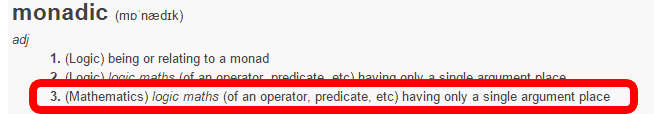To find the length of an array, you can use Rho $latex \rho$ monadically:

$latex Price \gets 1 \ 2 \ 3 \ 4 \ 5 \\* \rho Price$

=> 5

You can also Rho  $latex \rho$ dyadically to organize items into specified shapes, i.e.

$latex Tab \gets 4 \ 2 \ \rho \ 25 \ 60 \ 33 \ 47 \ 11 \ 44 \ 53 \ 28 \\* Tab$

=>

$latex 25 \ 60 \\* 33 \ 47 \\* 11 \ 44 \\* 53 \ 28$

where 4 2 describes the shape – 4 rows, 2 columns, followed by Rho $latex \rho$ and the array of items to be organized.

If the number of items in the array doesn’t match the number of slots in the shape we’re trying to organize into, then the array is repeated, e.g.

$latex 3 \ 2 \ \rho \ 1 \ 2 \ 3 \ 4$

=>

$latex 1 \ 2 \\* 3 \ 4 \\* 1 \ 2$

$latex 3 \ 2 \ \rho \ 1 \ 2$

=>

$latex 1 \ 2 \\* 1 \ 2 \\* 1 \ 2$

If there are too many items then extra items are ignored:

$latex 2 \ 2 \ \rho \ 1 \ 2 \ 3 \ 4 \ 5 \ 6$

=>

$latex 1 \ 2 \\* 3 \ 4$

Utilizing the way items are repeated, there’s a neat trick you can do, e.g.

$latex 3 \ 3 \ \rho \ 1 \ 0 \ 0 \ 0$

=>

$latex 1 \ 0 \ 0 \\* 0 \ 1 \ 0 \\* 0 \ 0 \ 1$

In general, APL has special names for data depending on its shape:

scalar – single value

vector – list of values

matrix – array with 2 dimensions

array – generic term for any set of values, regardless of no. of dimensions

table – common term for matrix

cube – common term for array with 3 dimensions

Ok, now that we’ve introduced the notion of dimensions, it’s time to confess that I slightly misled you earlier – Rho $latex \rho$ actually returns the shape of an array (not just its length, which only applies to a vector), so it works with multi-dimensional data too.

$latex \rho (2 \ 2 \ \rho \ 1 \ 2 \ 3\ 4)$

=> 2 2

Furthermore, since the result of Rho $latex \rho$ is itself a vector, applying Rho $latex \rho$ again will give us the number of dimensions an array has – otherwise known as its Rank.

$latex \rho \rho (2 \ 2 \ \rho \ 1 \ 2 \ 3 \ 4)$

=> 2

i.e. scalars have 0 rank, vectors have 1 rank, and so on…

You can also reduce over a set of values using / e.g. a plus reduction to sum up all the numbers in an array can be written as

$latex +/ \ 21 \ 45 \ 18 \ 27 \ 11$

=> $latex 122$

similarly, factorial can be written using multiply reduction:

$latex \times/ \ 1 \ 2 \ 3 \ 4 \ 5$

=> $latex 120$

The / symbol is an operator, whereas $latex + \ – \ \times \ \lceil$ etc. are functions. / can accept any of these functions and uses it to reduce over an array.

$latex \lceil/ \ 1 \ 3 \ 5 \ 7 \ 9 \ 6 \ 4 \ 2$

=> 9

$latex \lfloor/ \ 1 \ 3 \ 5 \ 7 \ 9 \ 6 \ 4 \ 2$

=> 1

APL calls programs defined functions, and you can create a defined function like this:

$latex Average \gets \{ (+/ \ \omega) \div \rho \omega \}\\* Average \ 1 \ 2 \ 3 \ 4 \ 5$

=> 3

where:

$latex Average$ – program name

$latex \omega$ – generic symbol for array passed on the right

$latex \alpha$ – generic symbol for array passed on the left

for example, if we have a function $latex f$ such that

$latex f \gets \{ (+/ \ \omega) – (+/ \ \alpha) \}\\* 1 \ 2 \ 3 \ f \ 4 \ 5 \ 6 \ 7$

=> 16

what we’ve done here is to sum the array on the right of $latex f$ – 4 5 6 7 – and subtract it with the sum of the array on the left – 1 2 3.

Simple, right? Let’s try a few more.

$latex Plus \gets \{ \omega + \alpha \}\\* Times \gets \{ \omega \times \alpha \}\\* (3 \ Plus \ 6) \ Times \ (2 \ Plus \ 5)$

=> 63

and you can use your custom functions with reduction:

$latex Plus/ \ 1 \ 2 \ 3 \ 4 \ 5$

=> 15

To index into an array, you can use the standard [ ] notation:

$latex Val \gets 1 \ 2 \ 3 \ 4 \ 5\\* Val$

=> 4

noticed that? APL is one-indexed!

What’s more, just like everything else, you can index into array with either a scalar or an array:

$latex Val[1 \ 3 \ 4]$

=> 1 3 4

$latex Val[1 \ 3 \ 4 \ 1]$

=> 1 3 4 1

This also works when it comes to updating values in an array:

$latex Val[1 \ 3 \ 5] \gets 42\\* Val$

=> 42 2 42 4 42

$latex Val[1 \ 3 \ 5] \gets 1 \ 3 \ 5\\* Val$

=> 1 2 3 4 5

$latex Val[2 \ 2 \ \rho \ 1 \ 2 \ 3 \ 4]$

=>

$latex 1 \ 2 \\* 3 \ 4$

Note that it follows the same rule as functions with regards to the input being either a scalar or a same-shaped array.

If the shape of the array doesn’t match, then it’ll error:

$latex Val[1 \ 3 \ 5] \gets 2 \ 4\\* Val$

=> $latex LENGTH \ ERROR$

Equally, if you use an invalid index, you’ll also get an error:

$latex Val$

=> $latex INDEX \ ERROR$

You can use Iota $latex \iota$ to generate an array of integers from 1 to N, e.g.

$latex \iota 4$

=> 1 2 3 4

$latex Val[\iota 4]$

=> 1 2 3 4

Booleans are represented as 1 and 0, and you can use any one of these relational functions: < = =/ > $latex \leq$ $latex \geq$

$latex 1 \ 3 \ 5 \ > \ 6 \ 2 \ 4$

=> 0 1 1

$latex 1 \ 3 \ 5 \ < \ 6 \ 2 \ 4$

=> 1 0 0

AND and OR semantics are represented by $latex \land$ and $latex \lor$ respectively.

$latex (1 \ 3 \ 5 > 6 \ 2 \ 4) \land (1 \ 3 \ 5 = 6 \ 3 \ 1)$

=> 1 0 1

$latex (1 \ 3 \ 5 > 6 \ 2 \ 4) \lor (1 \ 3 \ 5 = 6 \ 3 \ 1)$

=> 0 1 1

you can use them to count no. of employees based on salary for instance:

$latex Salaries \gets 1000 \ 1500 \ 2750 \ 3000 \ 2000\\* +/ (Salaries > 2500)$

=> 2

You can also use an array of boolean values as masks too:

$latex 1 \ 0 \ 1 \ / \ 1 \ 2 \ 3$

=> 1 3

$latex 1 \ 0 \ 1 \ / \ ‘iou’$

=> iu

this is called compression, and is useful for selecting items conforming to some criteria, e.g.

$latex Val \gets 11 \ 13 \ 1 \ 8 \ 9 \ 15 \ 7\\* Val > 10$

=> 1 1 0 0 0 1 0

$latex \rho Val$

=> 7

$latex \iota \rho\ Val$

=> 1 2 3 4 5 6 7

therefore, to find the indices of values that’s greater than 10

$latex (Val > 10) \ / \ \iota \rho Val$

=> 1 2 6

and to get the corresponding values, just use compression:

$latex (Val > 10) \ / \ Val$

=> 11 13 15

Given two arrays, A and B, you can return a new array with all the elements of A minus all the elements of B using the without operator ~

$latex A \gets 1 \ 2 \ 3\\* B \gets 3 \ 4 \ 5\\* A \sim B$

=> 1 2

this doesn’t modify A or B though.

$latex A$

=> 1 2 3

$latex B$

=> 3 4 5

memberships

To find items from an array that exists in another array, you can use the membership operator $latex \epsilon$

$latex (\iota 5) \ \epsilon \ 1 \ 3 \ 5$

=> 1 0 1 0 1

it also works with strings

$latex Phrase \gets \ ‘Panama \ is \ a \ canal \ between \ Atlantic \ and \ Pacific’\\* Phrase \ \epsilon \ ‘aeiouy’$

=> 0 1 0 1 0 1 0 1 0 0 1 0 0 1 0 1 0 0 0 1 0 0 1 1 0 0 0 0 0 1 0 0 1 0 0 1 0 0 0 0 1 0 1 0 1 0

you can (like before) get the indices of matches using compression:

$latex (Phrase \ \epsilon \ ‘aeiouy’) \ / \ \iota \rho Phrase$

=> 2 4 6 8 11 14 16 20 23 24 30 33 36 41 43 45

or find the matching characters using compression:

$latex (Phrase \ \epsilon \ ‘aeiouy’) \ / \ Phrase$

=> aaaiaaaeeeaiaaii

inspecting the result tells us that those are indeed the characters we’re looking for!

search

We saw how we can combine memberships and iota-rho $latex \iota \rho$ to find the indices in Phrase that corresponds to a member in ‘aeiouy’.

What if we want to find the first index each character in ‘aeiouy’ appears in Phrase, i.e. “aeiouy”.Select(char -> Phrase.IndexOf(char)) if you’re coming from a .Net background.

We can use the dyadic form of Iota $latex \iota$:

$latex Phrase \ \iota \ ‘aeiouy’$

=> 2 20 8 47 47 47

note that the length of Phrase is 46

$latex \rho Phrase$

=> 46

so the result 47 is essentially saying that ‘o’, ‘u’, ‘y’ never appeared in Phrase.

You can model an ‘else’ semantic using two arrays whose lengths are off by 1. For instance:

$latex Area \gets \ 17 \ 50 \ 59 \ 84 \ 89\\* Discount \gets \ 9 \ 8 \ 6 \ 5 \ 4 \ 2$

based on the way search works (not found = length + 1), we can map the specified Area codes to corresponding discount rates: 17 -> 9, 50 -> 8, … 89 -> 4, and all others to 2.

$latex D \gets \ 24 \ 75 \ 89 \ 60 \ 92 \ 50 \ 51 \ 50 \ 84 \ 66 \ 17 \ 89\\* Area \ \iota \ D$

=> 6 6 5 6 6 2 6 2 4 6 1 5

so where D does not exist in Area, you’ll get index 6 which does not exist in Area, BUT exists in Discount – i.e. the ‘other’ case we’re trying to model.

So if we use the result of $latex Area \ \iota \ D$ as indices in Discount we’ll find the corresponding discount rate for the values in D:

$latex Discount[Area \ \iota \ D]$

=> 2 2 4 2 2 8 2 8 5 2 9 4

this is an algorithm for changing the frame of reference, i.e. changing a list of area codes into a list of discount values, a general form can be described as:

$latex FinalSet[InitialSet \ \iota \ Values]$

where $latex \rho FinalSet$ = $latex \rho InitialSet$ + 1

take and drop

You can use the Take $latex \uparrow$ and Drop $latex \downarrow$ functions like you do with Enumerable.Take and Enumerable.Drop in LINQ:

$latex L \gets \iota 10\\* 4 \ \uparrow \ L$

=> 1 2 3 4

$latex 4 \ \downarrow \ L$

=> 5 6 7 8 9 10

if the count is negative then you take from the end of the list, e.g. take last 3 items or drop last 7 items:

$latex ^-3 \ \uparrow \ L$

=> 8 9 10

$latex ^-7 \ \downarrow \ L$

=> 1 2 3

Given a list of values

$latex L \gets 3 \ 8 \ 5 \ 14 \ 34 \ 5 \ 17 \ 21 \ 18$

suppose you want to find the change from one number to the next, i.e. 3 (+5) 8 (-3) 5 (+9) 14…

considering that

$latex 1 \ \downarrow \ L$

=> 8 5 14 34 5 17 21 18

$latex ^-1 \ \downarrow \ L$

=> 3 8 5 14 34 5 17 21

so now you can subtract the two arrays (of equal length) to get the answer:

$latex (1 \ \downarrow \ L) – (^-1 \ \downarrow \ L)$

=> $latex 5 \ ^-3 \ 9 \ 20 \ ^-29 \ 12 \ 4 \ ^-3$

clever, right? It’s reminiscent of the RX approach to tracking mouse moves.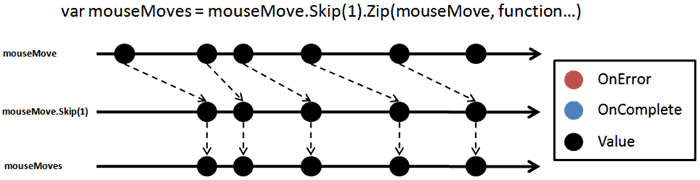mirrors and transposition

You can also pivot data about any direction easily: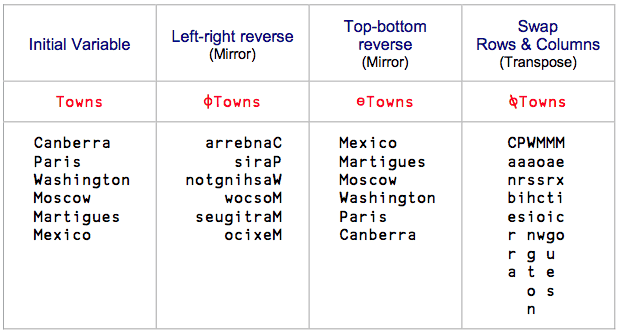(screenshot taken from Mastering Dyalog APL)

outer products

You can use the outer product operator $latex \circ.$ to calculate the cartesian products of two lists:

$latex 1 \ 2 \ 3 \ \circ.\times \ 3 \ 4 \ 5$

=>

$latex 3 \ 4 \ \ 5\\* 6 \ 8 \ \ 10\\* 9 \ 12 \ 15$

using the small circle + dot $latex \circ.$ + an operation to apply, in this case multiply $latex \times$, but could easily be anything else:

$latex 1 \ 2 \ 3 \ \circ.+ \ 3 \ 4 \ 5$

=>

$latex 4 \ 5 \ 6\\* 5 \ 6 \ 7\\* 6 \ 7 \ 8$

here’s even more examples taken from Mastering Dyalog APL: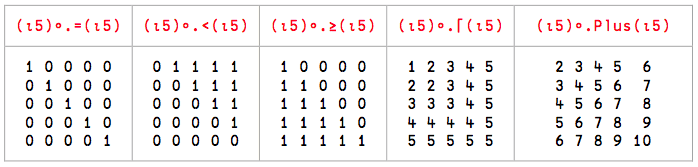Unlike some of the operations we’ve seen so far, the outer product operator works across different sized arrays too:

$latex 1 \ 2 \ 3 \ \circ.+ \ 3 \ 4 \ 5 \ 6$

=>

$latex 4 \ 5 \ 6 \ 7\\* 5 \ 6 \ 7 \ 8\\* 6 \ 7 \ 8 \ 9$

Finally, there are some common patterns (or idioms) you’ll see in APL.

• find indices in A that exists in B

$latex (A \ \epsilon \ B) \ / \ \iota \rho A$

• find the members (not indices) of A that exists in B

$latex (A \ \epsilon \ B) \ / \ A$

• find indices in A where B first appeared in (i.e. IndexOf)

$latex A \ \iota \ B$

• change the frame of reference

$latex FinalSet[InitialSet \ \iota \ Values]$

• generate indices with incrementing steps

$latex Start + Step \times (\iota Length) – 1$

So that’s it, I hope this post has given you a flavour of APL and help you learn the basics quickly. I find the array programming paradigm absolutely mind blowing and a completely different way to think about problems.

Also I find it has a similar transformative effect in changing the way I think about variables – as a point-in-time snapshot of some scalar value – to FRP (e.g. signals in Elm or observables in RX).

In the next post, let’s see how we can use APL to solve some simple problems (that’s all I’m able to manage for now!).

### 23 thoughts on “Fear and Loathing with APL”

1.Great introduction — I’ve always been curious about APL and this is a perfect taster!

2.theburningmonk

Thank you, Scott :-)

3.Very nice article and Good Luck with APL. If you want to “use APL to solve some sim­ple prob­lems” then a good place to start might be the competition questions at http://www.dyalog.com/student-competition.htm (this is an annual competition aimed at students who are new to APL; you can enter this year’s competition or look at some of the previous years). The Project Euler questions are also APL-solvable! There’s a complete set of documentation for Dyalog that can be downloaded free of charge at http://www.dyalog.com/documentation if you need any extra pointers.

I look forward to your next installment :-)

P.S. Re the Game of Life line at the top of your post – have you seen https://www.youtube.com/watch?v=a9xAKttWgP4 ?

4.Hi Fiona, I’ve not seen that video before, it’s great! I’ve updated the post to include the video at the top, I’m sure others will find it useful to see how the magic happens step by step too :-)

5.I have to say I quite enjoyed the read. In terms of real world applications I can’t think of one that I will ever have but its a quite cool language!

6.Thanks greg :-)

Same here, I don’t have any real world use cases in my line of work either but I do enjoy the opportunity for thought exercise. Fun to think about different ways to solve problems through only matrix manipulations!

Phil’s company (SimCorp) uses APL and they have a couple of full time APL developers there, so we can ask him what problems they are solving with APL next time.

7.I can imagine some problems it would work well on. I cannot imagine however that I would pick it over other more common/approachable alternatives. I love learning new things though and it looks to be fun to play with (and quite succinct once you wrap your head around the syntax).

8.For the right kinda problems it might be a good language to quickly prototype/experiment with given its succinct nature and ability to do array/matrix manipulation easily, and then convert to something more approachable – F# + Math.net perhaps.

9.As a long-time APLer, I’m lways excedited to see others getting on board and really enjoyed your article! ;-)

But it’s funny to see the debate abour “real world use cases” as there are infinite uses cases out there! Personally, I’m developing/selling an app for Sales Forecasting, Production Planning etc. which had been used i.e. by Kraft Foods in Germany for 15+ yrs (until they had to switch to SAP because of “IT-strategy”). APL gives you everything for modern real-world-apps (and, btw, it is getting more cross-plattform, too: Unix/Linux and Windows were always on the list, Dyalog (my favourite vendor) have recently added MacOS and are even supporting the Raspberry Pi – check out http://www.dyalog.com/blog/category/raspberry-pi/ for a bit more cool code!). Oh, and you surely can also develop web-apps with Dyalog’s MiServer!

10.Hello, great to hear someone who has commercial experience with APL!

A friend of mine’s company (SimCorp) is using APL commercially and I hear they might be one of the (if not the) biggest commercial users of APL, though I dunno what exactly they’re doing.

Whilst it’s true that there’s a potentially infinite number of real world use cases, I think the question is really “when would you prefer APL over something else”?

For something like Matlab and Mathematica, they’ve pivoted/marketed themselves towards certain use cases such as image or signal processing, and although you can probably do web-apps in them too it wouldn’t be the primary reason you switch to them.

11.This is a great tutorial. You translate the jargon and explain the semantics in a way that’s approachable to programmers used to other languages. And you’re absolutely correct that APL changes how you think of problems and data for the better.

As always, when talking about the potential in array-oriented programming, I feel the need to link this paper which blew my mind further, even though I only have a tenuous understanding of a lot of it: http://www.ccs.neu.edu/home/pete/pub/esop-2014.pdf (“An array-oriented language with static rank polymorphism”)

12.Thank you :-) and thanks for the link to the paper

13.BTW, showed this to a former-IBM colleague of mine (APL was his first language!) and he was impressed, but mentioned the bit about defined functions isn’t really idiomatic:
“That is very non-universal syntax. I don’t believe GNU-APL supports it yet (though by now it might). APL2 didn’t support it last I knew.” He went on to explain that it was a proposed syntax for years, but never made it into any implementations. Truthfully, I’ve never seen it used either; is it in Dyalog?

(He also brought up ?IO but agreed that it’s usually better to pretend that doesn’t exist. ;)

14.yup, that syntax is from Dyalog APL, which is the only APL implementation that I have looked at

15.Great introduction to APL! I’m a student that’s been trying to teach myself APL but it seems like every source I can find gets me half way before I get totally confused with what’s going on.
This definitely cleared up a lot of confusion ( especially with masking ).

I’m not sure if you’ve heard of the website “Learn x in y minutes” but I think this tutorial would fit that website perfectly!

16.Thanks! I love the Learn X in Y Minutes pages, have used them to help me learn a number of languages in the past.

I agree a Learn APL in Y Minutes page will be very useful instead. I’ll take a shot at putting together first draft, thanks for the suggestion!

17.This is fantastic! I learnt APL on an internship way back in 1979 (yup, golfball typewriters and special character sets) and then applied it usefully on boot camp when I joined IBM as a management trainee in 1981. Since then I’ve done no virtually no coing but have just started learning Python for fun. It’s incredibly frustrating to learn about ‘the wonderful way Python handles arrays with NumPy’ when we’re talking about things that take a single instruction in APL!

So, thank you for this very concise refresher course and excellent reminder of the good old days.

Bizarre to think that APL was conceived as a metaphor for learning math.

18. Pingback: Code or Language? – The Report

19.The AND example might have a mistake:

(1 3 5 > 6 2 4) ? (1 3 5 = 6 3 1)

I think the result should be: 0 1 0